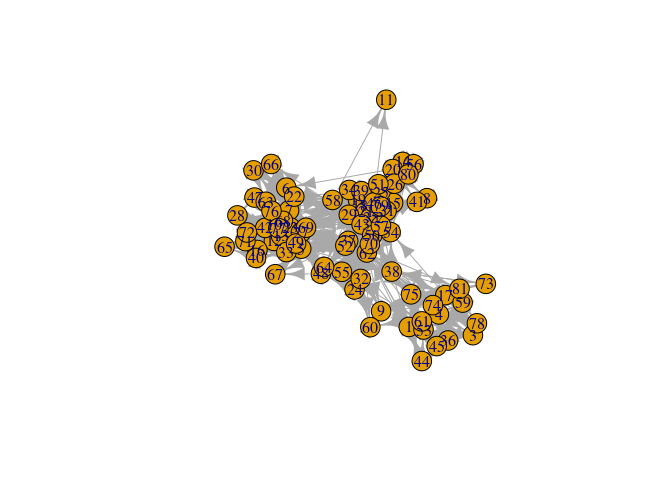# netplot

An alternative graph visualization engine that puts an emphasis on aesthetics at the same time of providing default parameters that provide visualizations that are out-of-the-box nice.

Some features:

1. Auto-scaling of vertices using sizes relative to the plotting device.
2. Embedded edge color mixer.
3. True curved edges drawing.
4. User-defined edge curvature.
5. Nicer vertex frame color.
6. Better use of space filling the plotting device.

The package uses the `grid` plotting system (just like `ggplot2`).

## Installation

And the development version from GitHub with:

``````# install.packages("devtools")
devtools::install_github("USCCANA/netplot")``````

## Example

This is a basic example which shows you how to solve a common problem:

``````library(igraph)
#>
#> Attaching package: 'igraph'
#> The following objects are masked from 'package:stats':
#>
#>     decompose, spectrum
#> The following object is masked from 'package:base':
#>
#>     union
library(netplot)
#>
#> Attaching package: 'netplot'
#> The following object is masked from 'package:igraph':
#>
#>     ego
set.seed(1)
data("UKfaculty", package = "igraphdata")
l <- layout_with_fr(UKfaculty)

plot(UKfaculty, layout = l) # ala igraph````````nplot(UKfaculty, layout = l) # ala netplot````sna::gplot(intergraph::asNetwork(UKfaculty), coord=l)``### UKfaculty

``````# Random names
set.seed(1)
nam <- sample(babynames::babynames\$name, vcount(UKfaculty))

ans <- nplot(
UKfaculty,
layout                = l,
vertex.color          = grDevices::hcl.colors(5, "Plasma")[V(UKfaculty)\$Group + 1],
vertex.label          = nam,
vertex.size.range     = c(.01, .04, 4),
vertex.label.col      =  "black",
vertex.label.fontface = "bold",
bg.col                = "transparent",
vertex.label.show     = .5,
vertex.label.range    = c(10, 25),
edge.width.range      = c(1, 4, 5)
)

# Plot it!
ans``````### USairports

``````# Loading the data
data(USairports, package="igraphdata")

# Generating a layout naively
layout   <- V(USairports)\$Position
layout   <- do.call(rbind, lapply(layout, function(x) strsplit(x, " ")[]))
layout[] <- stringr::str_remove(layout, "^[a-zA-Z]+")
layout   <- matrix(as.numeric(layout[]), ncol=2)

# Some missingness
layout[which(!complete.cases(layout)), ] <- apply(layout, 2, mean, na.rm=TRUE)

# Have to rotate it (it doesn't matter the origin)
layout <- netplot:::rotate(layout, c(0,0), pi/2)

# Simplifying the network
net <- simplify(USairports, edge.attr.comb = list(
weight = "sum",
name   = "concat",
Passengers = "sum",
"ignore"
))

# Getting a pretty color

f <- 1.5
rev(grDevices::hcl.colors(10, alpha = .5)),
red.f = f, blue.f = f, green.f = f
)

nplot(
net,
layout            = layout,
edge.width        = E(net)\$Passengers,
vertex.color      = col,
skip.vertex       = TRUE,
vertex.size.range = c(0,0),
edge.width.range  = c(.75, 4, 4),
bg.col            = "black"
)``````